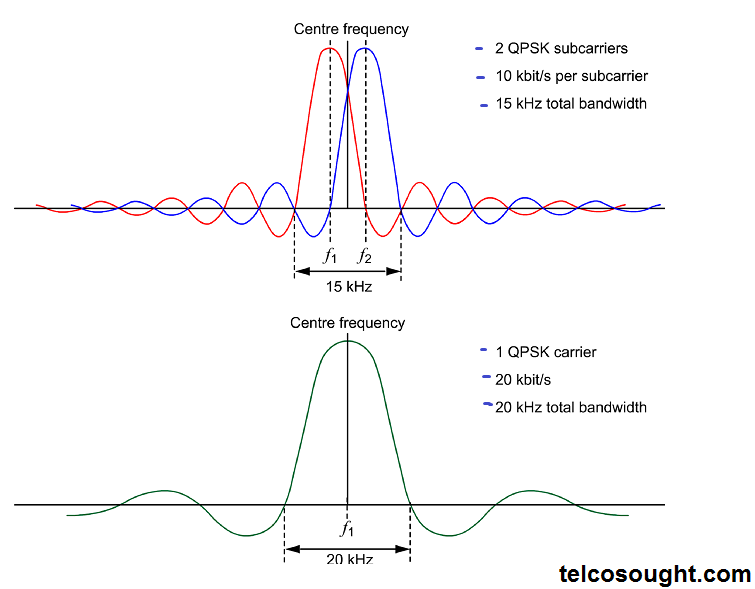# Radio Carrier Orthogonality- Ofdm Principles

In this 4G ran article we learn about the OFDM Principles-  Radio Carrier Orthogonality and Spectral Efficiency in OFDM.

Radio Carrier Orthogonality Think about a radio transmitter being modulated with 10 kbit/s bit-stream with QPSK (Quadrature Phase Shift Keying). You could observe a spectral envelope as a result of a (sin x)(sin x) / x function as illustrated in the diagram.

Spectral Efficiency in OFDM When we consider the two interlocking two QPSK radio carrier carriers it is observed it has high spectral efficiency. If the actual bandwidth of the transmitting signal is thought to be the frequency separation between the nulls that are first, then the single QPSK carrier modulated at 10 kbit/s would result in the null-to-null bandwidth in the range of 10 kHz.

Think about a radio transmitter being modulated with 10 kbit/s bit-stream with QPSK (Quadrature Phase Shift Keying). You could observe a spectral envelope as a result of a (sin x)(sin x) / x function as illustrated in the diagram. The first null is located 5 kHz away from the center frequency.

In a traditional FDM (Frequency Division Multiplexing) system, additional radio carriers are allocated and separated from the primary one to ensure that there is no interference between adjacent channels. In terms of size, the required guard band will be determined by the characteristics of the receiver and transmitter as well as their relative strengths.

In this system, it is assumed there isn’t any synchronization between the possible interference sources. This is what results in the requirement for a large frequency gap between carriers. If there were the possibility of synchronization between channels and channels, a smaller time-to-flight could also be utilized. The trick is being in a position to utilize the intricate nature of the instantly transmitted spectrum. The modulation envelope is an artificial method of indicating the possibilities of time. the snapshot of an instant in time could look very different.

Take a second radio carrier assigned in a way that its frequency of operation coincides precisely with that of the zero of the envelope of the first. It’s using the same modulation scheme and has exactly the same rate of data. The result is as follows. Notice that the spacing of the carriers of 5 kHz has the same as the symbol speed in 5kbps. The spectra of both carriers are now overlapping but, so long as the frequencies of the carriers and baseband data are precisely synchronized, they can be successfully demodulated. The reason for this is that the relationship between the offset of the center frequency and symbol rate keeps an extremely high degree of orthogonality between both radio carriers.

## Spectral Efficiency in OFDM

When we consider the two interlocking two QPSK radio carrier carriers it is observed it has a high spectral efficiency. If the actual bandwidth of the transmitting signal is thought to be the frequency separation between the nulls that are first, then the single QPSK carrier modulated at 10 kbit/s would result in the null-to-null bandwidth in the range of 10 KHz.There are two sub-carriers, each which can carry 10 kbit/s of QPSK. The null-to-null spectrums of both sub-carriers overlap with 5 kHz. This results in a null-to-null bandwidth for the carriers of 15 KHz. Therefore, QPSK can transport 20 kbit/s over the frequency range that is 15 kHz. Be aware that a single QPSK-modulated carrier with 20 kbit/s could yield a null-tonull bandwidth in the range of 20 KHz.

The principle of receiving independent of orthogonal radio transmitters with overlap spectrums is extended by utilizing a larger variety of radio carriers with narrow bands within a single wideband channel allocation. This produces a effective spectrally efficient channel which can be able to carry very high bits.

For instance, if 1000 orthogonal radios were modulated by QPSK and each carried 10 kbit/s of data, the total capacity of this channel is 10Mbps. This would mean an overall channel bandwidth slightly greater than 5 MHz. The same bit rate as QPSK modulation on the same radio carrier will need a null-to-null bandwith of 10 MHz. This means that OFDM (Orthogonal Frequency Division Multiplexing) nearly increases the efficiency of spectral. Additionally, the OFDM transmission will be much more resistant to multipath effects within the channel.

Also Read:-  EPS UE BEARERS AND CONNECTIVITY